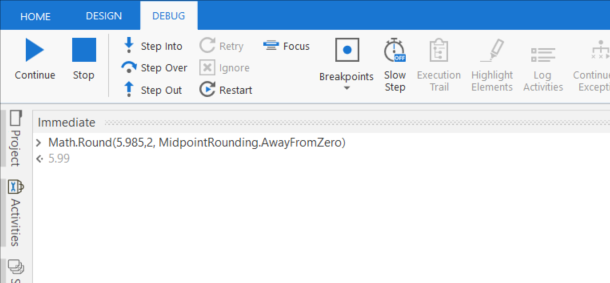# Rounded from 5 to higher

Hello, I used this formula `Math.Round(((Var1*Var2), 2)` to round up to the second decimal but the problem is that when the product of Var1 by Var2 ends for example 5.985, the formula rounds me to 5.98 instead of 5.99.
Thank you

1 Like

Hi,

Can you try the following?

``````Math.Round( 5.985, 2,MidpointRounding.AwayFromZero)
``````

Regards,

give a try on`Math.Round(Var1*Var2,2, MidpointRounding.AwayFromZero)`

It works, thank so much

This topic was automatically closed 3 days after the last reply. New replies are no longer allowed.